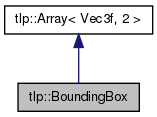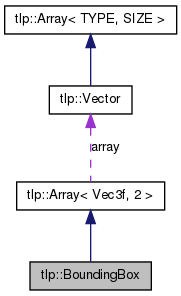Overview Modules Class Hierarchy Classes Members
tlp::BoundingBox Struct Reference

This class represents the 3D bounding box of an object. It is mostly used to determine whether or not two object are in a state of collision. More...

`#include <BoundingBox.h>`

Inheritance diagram for tlp::BoundingBox:[legend]
Collaboration diagram for tlp::BoundingBox:[legend]

List of all members.

## Public Member Functions

• BoundingBox ()
Creates an invalid boundig box. The minimum is (1, 1, 1) and the maximum is (-1, -1, -1).
• BoundingBox (const tlp::Vec3f &min, const tlp::Vec3f &max)
Creates a bounding box that must be valid. Validity is checked in debug mode by an assert.
• Vec3f center () const
Returns the geometrical center of the bounding box. An assertion is raised in debug mode if the BoundingBox is not valid.
• float width () const
Returns the width of the bounding box An assertion is raised in debug mode if the BoundingBox is not valid.
• float height () const
Returns the height of the bounding box An assertion is raised in debug mode if the bounding box is not valid.
• float depth () const
Returns the depth of the bounding box An assertion is raised in debug mode if the bounding box is not valid.
• void expand (const tlp::Vec3f &coord)
Expands the bounding box to one containing the vector passed as parameter. If the parameter is inside the bounding box, it remains unchanged.
• void translate (const tlp::Vec3f &vec)
Translates the bounding box by the displacement given by the vector passed as parameter.
• bool isValid () const
Checks whether the bounding box's lowest point is less than it's highest point. "Less Than" means axis-by-axis comparison, i.e. x1 < x2 && y1 < y2 && z1 < z2.
• bool contains (const tlp::Vec3f &coord) const
Checks if the given vector is inside the current bounding box. If the bounding box is invalid the result is always false.
• bool intersect (const tlp::BoundingBox &boundingBox) const
Checks if the given bounding box intersect the current one. If one of the bounding box is invalid return false.
• bool intersect (const Vec3f &segStart, const Vec3f &segEnd) const
Checks if the bounding box intersects a given line segment. If the bounding box is invalid the result is always false.
• void getCompleteBB (Vec3f bb) const
The vector passed as parameter is modified to contain the 8 points of the bounding box. The points are, in order : 0: lower leftmost closest point (the bounding box's minimum) 1: lower rightmost closest point 2: highest rightmost closest point 3: highest leftmost closest point 4: lower rightmost farthest point 5: lower rightmost farthest point 6: highest rightmost farthest point 7: highest leftmost farthest point.

## Detailed Description

This class represents the 3D bounding box of an object. It is mostly used to determine whether or not two object are in a state of collision.

It is defined by two 3d points, the first one (A) being the lowest point, the second (B) being the highest. As a bounding box is a mathematical entity describing the lowest and highest points, whether these points are in the top-left corner or lower-right corner depends on the axes we use. Below is a crude ASCII-art description of the axes we use in our 3D world and the points where the min and max are thus positioned. Through the rest of this class's documentation, it will be assumed that this is the convention.

y | | |_____ x / / z

_________ B / /| / / | /________/ | | | | | | | | | / |________|/ A

Author : Tulip team

## Constructor & Destructor Documentation

 tlp::BoundingBox::BoundingBox ( )

Creates an invalid boundig box. The minimum is (1, 1, 1) and the maximum is (-1, -1, -1).

 tlp::BoundingBox::BoundingBox ( const tlp::Vec3f & min, const tlp::Vec3f & max )

Creates a bounding box that must be valid. Validity is checked in debug mode by an assert.

Parameters:
 min The lower left closest point of the box. max The higher left most farther point of the box.

## Member Function Documentation

 Vec3f tlp::BoundingBox::center ( ) const

Returns the geometrical center of the bounding box. An assertion is raised in debug mode if the BoundingBox is not valid.

Returns:
The center of the bounding box :Vec3f
 bool tlp::BoundingBox::contains ( const tlp::Vec3f & coord ) const

Checks if the given vector is inside the current bounding box. If the bounding box is invalid the result is always false.

Parameters:
 coord A point in the 3D space.
Returns:
bool Wether coord is in the bounding box.
 float tlp::BoundingBox::depth ( ) const

Returns the depth of the bounding box An assertion is raised in debug mode if the bounding box is not valid.

 void tlp::BoundingBox::expand ( const tlp::Vec3f & coord )

Expands the bounding box to one containing the vector passed as parameter. If the parameter is inside the bounding box, it remains unchanged.

Parameters:
 coord A point in the 3D space we want the bounding box to encompass.
Returns:
void
 void tlp::BoundingBox::getCompleteBB ( Vec3f bb ) const

The vector passed as parameter is modified to contain the 8 points of the bounding box. The points are, in order : 0: lower leftmost closest point (the bounding box's minimum) 1: lower rightmost closest point 2: highest rightmost closest point 3: highest leftmost closest point 4: lower rightmost farthest point 5: lower rightmost farthest point 6: highest rightmost farthest point 7: highest leftmost farthest point.

Crude ASCII art again, sorry for your eyes.

6_________ 7 /| /| / | / | 3/__|_____/2 | | |_____|__| | /4 | /5 | / | / |/_______|/ 0 1

Parameters:
 bb A vector in which to put the points of the bounding box.
Returns:
void
 float tlp::BoundingBox::height ( ) const

Returns the height of the bounding box An assertion is raised in debug mode if the bounding box is not valid.

 bool tlp::BoundingBox::intersect ( const tlp::BoundingBox & boundingBox ) const

Checks if the given bounding box intersect the current one. If one of the bounding box is invalid return false.

Parameters:
 boundingBox The bounding box to compare with.
Returns:
bool Wether the bounding boxes intersect.
 bool tlp::BoundingBox::intersect ( const Vec3f & segStart, const Vec3f & segEnd ) const

Checks if the bounding box intersects a given line segment. If the bounding box is invalid the result is always false.

Parameters:
 segStart the start point of the line segment on which to check intersection segEnd the end point of the line segment on which to check intersection
Returns:
bool Wether the line segment intersects the bounding box
 bool tlp::BoundingBox::isValid ( ) const

Checks whether the bounding box's lowest point is less than it's highest point. "Less Than" means axis-by-axis comparison, i.e. x1 < x2 && y1 < y2 && z1 < z2.

Returns:
bool Whether this bounding box is valid.
 void tlp::BoundingBox::translate ( const tlp::Vec3f & vec )

Translates the bounding box by the displacement given by the vector passed as parameter.

Parameters:
 vec The displacement vector in 3D space to translate this bounding box by.
Returns:
void
 float tlp::BoundingBox::width ( ) const

Returns the width of the bounding box An assertion is raised in debug mode if the BoundingBox is not valid.

 Tulip Software by LaBRI Visualization Team    2001 - 2012